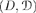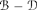# 2.1. Generalities

The examples given in Chapter 1 show that a statistical problem may be characterized by the following elements:

a probability distribution that is not entirely known;

observations;

Formalization: Wald’s decision theory provided a common framework for statistics problems. We take:

1) A tripletwhere P is a family of probabilities on the measurable spaceis called a statistical model. We often set P = {Pθ, θ ∈ Θ} where we suppose thatis injective, and Θ is called the parameter space.

2) A measurable spaceis called a decision (or action) space.

3) A setof measurable mappingsis called a set of decision functions (d.f.) (or decision rules).

Description: From an observation that follows an unknown distribution P ∈ P, a statistician chooses an element aD using an element d from .

Preference relation ...

Get Mathematical Statistics and Stochastic Processes now with the O’Reilly learning platform.

O’Reilly members experience books, live events, courses curated by job role, and more from O’Reilly and nearly 200 top publishers.